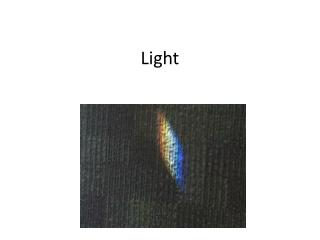DownloadDownload PresentationLight

# Light

Download Presentation## Light

- - - - - - - - - - - - - - - - - - - - - - - - - - - E N D - - - - - - - - - - - - - - - - - - - - - - - - - - -
##### Presentation Transcript

1. Light

2. Previous knowledge • Tell me one of your experience or vocabulary about light.

3. What is light? energy • Light is _______. • Light travels in a _______ . • Light consists of particles called _________. wave photons

4. Light Spectrum • White light can be separated into 6 colors. • The lowest frequency of light we can see with our eyes appears ______. • The highest frequency of light appear _______. red violet

5. Electromagnetic Waves(E=hf) What characteristics of light determines the amount of energy carried by a photon of the light? Frequency

6. E=hf • What is the frequency of a photon which has 4.41 x 10-19 J of energy? (Planck’s constant is 6.63 x 10-34 kgm2/s) • E=hf • Answer: 6.65 x 1014 Hz

7. Characteristics of light

8. Transparent vs. Opaque • Transparent: Materials that allow light to pass through. Ex) water, glass, atmosphere • Opaque: Materials that do not allow light to pass through. Ex) wood, stone, metal, people

9. X-rays Which part of a hand is opaque to x-rays? Skin or bone Which part of a hand is transparent to x-rays? Skin or bone

10. The Condition of Transparency • A chain of absorption and re-emission

11. Speed of light • Light travels at the speed of 3.00 x 108 m/s. • With this speed, light can travel from the sun to Earth in 8 minutes (about 500 seconds). • It takes 4 years for light to travel from the sun to the next nearest star in our galaxy. • Our galaxy has a diameter of 100,000 light-years.

12. v = f • λ • What is the frequency of a EM wave if it is a wavelength of 1000m? • 3.00 x 108 m/s = f • 1000 • 3.00 x 105 Hz = f

13. v = f • λ • The AM radio station sends a radio wave of 1.0 x 106 Hz. What is the wavelength of this wave? • 3.0 x 108 m/s = 1.0 x 106 • λ • 3.0 x 102 m =λ

14. Light slows down in glass or water. In water light travels at 75% of its speed or 0.75 c.

15. Wave Interactions • Waves can refract, or bend, as they change media.

16. Index of refraction • n = c/v • Index of refraction = speed of light in vacuum over speed of light in medium • n=1.333 (in water) • n=2.419 (in diamond)

17. Snell’s law • ni(sin θi) = nr(sin θr) • Index of refraction of first medium x sine of the angle of incidence = index of refraction of second medium x sine of the angle of refraction θi θr

18. Practice of Snell’s Law • A light ray of wavelength 589 nm traveling through air strikes a smooth, flat slab of crown glass at an angle of 30.0 degrees to the normal. Find the angle of refraction. • (nair=1.00, nglass=1.52) • Answer: 19.2 degrees

19. Critical Angle • At the critical angle, light beam no longer emerges into the air above the surface.

20. The Critical Angle in Sound

21. Wave Interactions

22. Previous experience • How can you look at the back of your head?

23. Flat mirrors • Light always travels in a straight line through a uniform substance. • If the surface is opaque, the light is deflected at the surface. • The change in the direction of the light is called reflection. • Specular reflection: reflected in one direction. • Diffuse reflection: reflected in many different directions

24. Virtual image

25. Reflection

26. If a straight line is drawn perpendicular to the reflecting surface at the point when the incoming ray strikes the surface, the angle of incidence and the angle of reflection can be defined with respect to the line.

27. θ = θ’ • Angle of incoming light ray = angle of reflected light ray

28. Object distance, image distance • The relationship between object distance from the mirror which is represented as p, and image distance, which is represented as q, is such that the object and image distance are equal. • The image formed by rays that appear to come together at the image point behind the mirror – but never really do – is called a virtual image.

29. p (object distance) = q (image distance) • h (object height) = h’ (image height) p q h h’

30. Polarization http://www.physicsclassroom.com/class/light/u12l1e.cfm for further information.

31. Linear polarization The process that separate waves with electric-field oscillations in the vertical direction from those in the horizontal direction, producing a beam of light with electric-field waves oriented in the same direction.